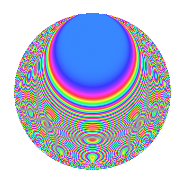# Properties

 Label 170.2.rLevel $170$ Weight $2$ Character orbit 170.r Rep. character $\chi_{170}(23,\cdot)$ Character field $\Q(\zeta_{16})$ Dimension $72$ Newform subspaces $2$ Sturm bound $54$ Trace bound $1$

# Related objects

## Defining parameters

 Level: $$N$$ $$=$$ $$170 = 2 \cdot 5 \cdot 17$$ Weight: $$k$$ $$=$$ $$2$$ Character orbit: $$[\chi]$$ $$=$$ 170.r (of order $$16$$ and degree $$8$$) Character conductor: $$\operatorname{cond}(\chi)$$ $$=$$ $$85$$ Character field: $$\Q(\zeta_{16})$$ Newform subspaces: $$2$$ Sturm bound: $$54$$ Trace bound: $$1$$ Distinguishing $$T_p$$: $$3$$

## Dimensions

The following table gives the dimensions of various subspaces of $$M_{2}(170, [\chi])$$.

Total New Old
Modular forms 248 72 176
Cusp forms 184 72 112
Eisenstein series 64 0 64

## Trace form

 $$72q + O(q^{10})$$ $$72q - 24q^{10} - 48q^{15} - 16q^{25} + 48q^{27} - 16q^{28} - 32q^{31} + 32q^{33} + 32q^{34} - 32q^{37} - 64q^{39} - 40q^{41} - 48q^{42} - 32q^{50} - 32q^{52} + 32q^{53} - 16q^{55} - 144q^{57} - 64q^{59} + 48q^{60} - 48q^{62} - 48q^{63} + 32q^{67} + 32q^{70} + 32q^{71} + 40q^{72} + 120q^{73} + 40q^{74} - 48q^{75} + 48q^{77} + 64q^{78} - 32q^{79} + 16q^{80} + 96q^{81} + 64q^{82} + 32q^{83} + 144q^{85} + 32q^{86} + 176q^{87} + 32q^{88} + 48q^{90} + 96q^{91} + 16q^{92} - 16q^{93} - 80q^{95} - 32q^{97} + 72q^{98} + 96q^{99} + O(q^{100})$$

## Decomposition of $$S_{2}^{\mathrm{new}}(170, [\chi])$$ into newform subspaces

Label Dim. $$A$$ Field CM Traces $q$-expansion
$$a_2$$ $$a_3$$ $$a_5$$ $$a_7$$
170.2.r.a $$32$$ $$1.357$$ None $$0$$ $$0$$ $$0$$ $$0$$
170.2.r.b $$40$$ $$1.357$$ None $$0$$ $$0$$ $$0$$ $$0$$

## Decomposition of $$S_{2}^{\mathrm{old}}(170, [\chi])$$ into lower level spaces

$$S_{2}^{\mathrm{old}}(170, [\chi]) \cong$$ $$S_{2}^{\mathrm{new}}(85, [\chi])$$$$^{\oplus 2}$$